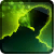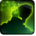# Virulent ToxinConditions

Used by

## Related effects, buffs and debuffs

Please click on an effect below to view its details.

• [0s]Virulent Toxin

 Slot: Other (not visible) Duration: instant # occurrences: 0 Tags: tag.abl.debuff.damage_over_time, tag.abl.debuff.poison Initializers: Set type to Other, cannot toggle off Conditions: If TARGET does not have any effect with the tag tag.​abl.​uprisings.​station256.​virulent_toxin Target overrides: [target override] Random Enemy- Ignore Current = (bool) true- Ignore NPC = (bool) false- Ignore PC = (bool) false- Ignore CC = (bool) false Global ID: 16140937807312690127 FQN: abl.exp.uprisings.station256.trial_and_error.boss_3.virulent_toxin/13/1
• 1
• When effect is applied

Perform the following actions:

• Play appearance epp.agent.toxic_dart.toxic_dart_real
• Invoke Projectile
- Determine Hit = (bool) false
- Timed Projectile = (bool) false
- Hit Checked = (bool) false
- Is Special Ability = (bool) false
- Ignore Dual Wield Modifier = (bool) false
- Slot = (int) 9
- Spell Type = (int) 1
- Travel Speed = (float) 4
- Travel Time = (time) 0s
• 2
• When projectile completed travel and hit target

Only when the following conditions are met:

• Is Polymorphed
- Negated = (bool) false
- Actor = (int) 3

Perform the following actions:

• Play appearance epp.exp.excavation.roth.multi.arcanum.rakghoul_bite_poison_rupture
• Spell Damage
- Disable Crit Roll = (bool) false
- Damage Type = (int) 4
- Slot = (int) 1
- Spell Type = (int) 2
- Level Cap = (int) 0
- Amount Max = (float) 0
- Amount Min = (float) 0
- Amount Percent = (float) 0
- Threat Percent = (float) 0
- Standard Health Percent Max = (float) 0.72
- Standard Health Percent Min = (float) 0.68
- Amount Modifier Percent = (float) 0
- Coefficient = (float) 0
- Health Steal Percentage = (float) 0
- Standard Rating Percent Min = (float) 0
- Standard Rating Percent Max = (float) 0
• 3
• When projectile completed travel and hit target

Only when the following conditions are met:

• Is Polymorphed
- Negated = (bool) true
- Actor = (int) 3

Perform the following actions:

• Play appearance epp.exp.excavation.roth.multi.arcanum.rakghoul_bite_poison_rupture
• Spell Damage
- Disable Crit Roll = (bool) false
- Damage Type = (int) 4
- Slot = (int) 1
- Spell Type = (int) 2
- Level Cap = (int) 0
- Amount Max = (float) 0
- Amount Min = (float) 0
- Amount Percent = (float) 0
- Threat Percent = (float) 0
- Standard Health Percent Max = (float) 0.2
- Standard Health Percent Min = (float) 0.16
- Amount Modifier Percent = (float) 0
- Coefficient = (float) 0
- Health Steal Percentage = (float) 0
- Standard Rating Percent Min = (float) 0
- Standard Rating Percent Max = (float) 0
• Add effect #2 to TARGET from CASTER, Combat Math Only
• [50s]Virulent Toxin (Physical)
Periodic internal damage that becomes more lethal with time.

 Slot: Debuff Duration: 50s On death: Effect is removed Tick rate: 5s (10 ticks) # occurrences: 1 Tags: tag.abl.uprisings.station256.virulent_toxin, tag.abl.debuff.damage_over_time, tag.abl.dispel_type.physical, tag.abl.debuff.poison, tag.abl.dispel_type.purge_only Initializers: Set Duration- Is Hidden = (bool) false- Is Real Time = (bool) false- Is Short Duration Persist = (bool) false- Toughness = (int) 1- Duration = (time) 50s- Max Duration = (time) 50sSet type to Negative, cannot toggle offSet Stack Limit- Is By Tag = (bool) true- Is Per Caster = (bool) true- Is Multi Target = (bool) false- Relevant Tags = (string) tag.abl.agent.corrosive_dart_dupe- Max Stack Count = (int) 1 Conditions: Global ID: 16140938906824317362 Combat log ID: 4088469563375894 FQN: abl.exp.uprisings.station256.trial_and_error.boss_3.virulent_toxin/13/2
• 1
• When effect is applied

Perform the following actions:

• Play appearance epp.exp.excavation.roth.multi.arcanum.rakghoul_bite_poison_loop, dependent on calling effect
• 2
• When effect ticks the 1st time

Perform the following actions:

• Spell Damage
- Disable Crit Roll = (bool) false
- Damage Type = (int) 4
- Slot = (int) 1
- Spell Type = (int) 2
- Level Cap = (int) 0
- Amount Max = (float) 0
- Amount Min = (float) 0
- Amount Percent = (float) 0
- Threat Percent = (float) 0
- Standard Health Percent Max = (float) 0.1
- Standard Health Percent Min = (float) 0.1
- Amount Modifier Percent = (float) 0
- Coefficient = (float) 0
- Health Steal Percentage = (float) 0
- Standard Rating Percent Min = (float) 0
- Standard Rating Percent Max = (float) 0
• 3
• When effect ticks the 2nd time

Perform the following actions:

• Play appearance epp.exp.excavation.roth.multi.arcanum.rakghoul_bite_poison_tick
• Spell Damage
- Disable Crit Roll = (bool) false
- Damage Type = (int) 4
- Slot = (int) 1
- Spell Type = (int) 2
- Level Cap = (int) 0
- Amount Max = (float) 0
- Amount Min = (float) 0
- Amount Percent = (float) 0
- Threat Percent = (float) 0
- Standard Health Percent Max = (float) 0.22
- Standard Health Percent Min = (float) 0.22
- Amount Modifier Percent = (float) 0
- Coefficient = (float) 0
- Health Steal Percentage = (float) 0
- Standard Rating Percent Min = (float) 0
- Standard Rating Percent Max = (float) 0
• 4
• When effect ticks the 3rd time

Perform the following actions:

• Play appearance epp.exp.excavation.roth.multi.arcanum.rakghoul_bite_poison_tick
• Spell Damage
- Disable Crit Roll = (bool) false
- Damage Type = (int) 4
- Slot = (int) 1
- Spell Type = (int) 2
- Level Cap = (int) 0
- Amount Max = (float) 0
- Amount Min = (float) 0
- Amount Percent = (float) 0
- Threat Percent = (float) 0
- Standard Health Percent Max = (float) 0.38
- Standard Health Percent Min = (float) 0.38
- Amount Modifier Percent = (float) 0
- Coefficient = (float) 0
- Health Steal Percentage = (float) 0
- Standard Rating Percent Min = (float) 0
- Standard Rating Percent Max = (float) 0
• 5
• When effect ticks the 4th time

Perform the following actions:

• Play appearance epp.exp.excavation.roth.multi.arcanum.rakghoul_bite_poison_tick
• Spell Damage
- Disable Crit Roll = (bool) false
- Damage Type = (int) 4
- Slot = (int) 1
- Spell Type = (int) 2
- Level Cap = (int) 0
- Amount Max = (float) 0
- Amount Min = (float) 0
- Amount Percent = (float) 0
- Threat Percent = (float) 0
- Standard Health Percent Max = (float) 0.58
- Standard Health Percent Min = (float) 0.58
- Amount Modifier Percent = (float) 0
- Coefficient = (float) 0
- Health Steal Percentage = (float) 0
- Standard Rating Percent Min = (float) 0
- Standard Rating Percent Max = (float) 0
• 6
• When effect ticks the 5th time

Perform the following actions:

• Play appearance epp.exp.excavation.roth.multi.arcanum.rakghoul_bite_poison_tick
• Spell Damage
- Disable Crit Roll = (bool) false
- Damage Type = (int) 4
- Slot = (int) 1
- Spell Type = (int) 2
- Level Cap = (int) 0
- Amount Max = (float) 0
- Amount Min = (float) 0
- Amount Percent = (float) 0
- Threat Percent = (float) 0
- Standard Health Percent Max = (float) 0.82
- Standard Health Percent Min = (float) 0.82
- Amount Modifier Percent = (float) 0
- Coefficient = (float) 0
- Health Steal Percentage = (float) 0
- Standard Rating Percent Min = (float) 0
- Standard Rating Percent Max = (float) 0
• 7
• When effect ticks the 6th time

Perform the following actions:

• Play appearance epp.exp.excavation.roth.multi.arcanum.rakghoul_bite_poison_tick
• Spell Damage
- Disable Crit Roll = (bool) false
- Damage Type = (int) 4
- Slot = (int) 1
- Spell Type = (int) 2
- Level Cap = (int) 0
- Amount Max = (float) 0
- Amount Min = (float) 0
- Amount Percent = (float) 0
- Threat Percent = (float) 0
- Standard Health Percent Max = (float) 1.1
- Standard Health Percent Min = (float) 1.1
- Amount Modifier Percent = (float) 0
- Coefficient = (float) 0
- Health Steal Percentage = (float) 0
- Standard Rating Percent Min = (float) 0
- Standard Rating Percent Max = (float) 0
• 8
• When effect ticks the 7th time

Perform the following actions:

• Play appearance epp.exp.excavation.roth.multi.arcanum.rakghoul_bite_poison_tick
• Spell Damage
- Disable Crit Roll = (bool) false
- Damage Type = (int) 4
- Slot = (int) 1
- Spell Type = (int) 2
- Level Cap = (int) 0
- Amount Max = (float) 0
- Amount Min = (float) 0
- Amount Percent = (float) 0
- Threat Percent = (float) 0
- Standard Health Percent Max = (float) 1.42
- Standard Health Percent Min = (float) 1.42
- Amount Modifier Percent = (float) 0
- Coefficient = (float) 0
- Health Steal Percentage = (float) 0
- Standard Rating Percent Min = (float) 0
- Standard Rating Percent Max = (float) 0
• 9
• When effect ticks the 8th time

Perform the following actions:

• Play appearance epp.exp.excavation.roth.multi.arcanum.rakghoul_bite_poison_tick
• Spell Damage
- Disable Crit Roll = (bool) false
- Damage Type = (int) 4
- Slot = (int) 1
- Spell Type = (int) 2
- Level Cap = (int) 0
- Amount Max = (float) 0
- Amount Min = (float) 0
- Amount Percent = (float) 0
- Threat Percent = (float) 0
- Standard Health Percent Max = (float) 1.78
- Standard Health Percent Min = (float) 1.78
- Amount Modifier Percent = (float) 0
- Coefficient = (float) 0
- Health Steal Percentage = (float) 0
- Standard Rating Percent Min = (float) 0
- Standard Rating Percent Max = (float) 0
• 10
• When effect ticks the 9th time

Perform the following actions:

• Play appearance epp.exp.excavation.roth.multi.arcanum.rakghoul_bite_poison_tick
• Spell Damage
- Disable Crit Roll = (bool) false
- Damage Type = (int) 4
- Slot = (int) 1
- Spell Type = (int) 2
- Level Cap = (int) 0
- Amount Max = (float) 0
- Amount Min = (float) 0
- Amount Percent = (float) 0
- Threat Percent = (float) 0
- Standard Health Percent Max = (float) 2.18
- Standard Health Percent Min = (float) 2.18
- Amount Modifier Percent = (float) 0
- Coefficient = (float) 0
- Health Steal Percentage = (float) 0
- Standard Rating Percent Min = (float) 0
- Standard Rating Percent Max = (float) 0
• 11
• When effect ticks the 10th time

Perform the following actions:

• Play appearance epp.exp.excavation.roth.multi.arcanum.rakghoul_bite_poison_tick
• Spell Damage
- Disable Crit Roll = (bool) false
- Damage Type = (int) 4
- Slot = (int) 1
- Spell Type = (int) 2
- Level Cap = (int) 0
- Amount Max = (float) 0
- Amount Min = (float) 0
- Amount Percent = (float) 0
- Threat Percent = (float) 0
- Standard Health Percent Max = (float) 2.62
- Standard Health Percent Min = (float) 2.62
- Amount Modifier Percent = (float) 0
- Coefficient = (float) 0
- Health Steal Percentage = (float) 0
- Standard Rating Percent Min = (float) 0
- Standard Rating Percent Max = (float) 0
• [hidden] [1.5s]Effect #3

 Slot: Debuff Duration: 1.5s On death: Effect is removed # occurrences: 0 Initializers: Set Duration- Is Hidden = (bool) false- Is Real Time = (bool) false- Is Short Duration Persist = (bool) false- Toughness = (int) 3- Duration = (time) 1.5s- Max Duration = (time) 1.5s Target overrides: [target override] Caster Global ID: 16140940006335944549 FQN: abl.exp.uprisings.station256.trial_and_error.boss_3.virulent_toxin/13/3
• 1
• When effect is applied

Perform the following actions:

• Silence